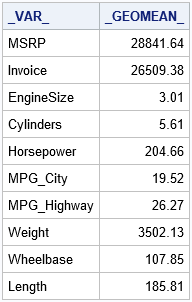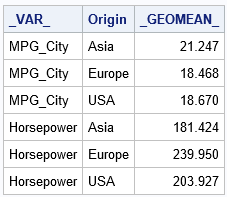I recently wrote about how to use PROC TTEST in SAS/STAT software to compute the geometric mean and related statistics. This prompted a SAS programmer to ask a related question. Suppose you have dozens (or hundreds) of variables and you want to compute the geometric mean of each. What is the best way to obtain these geometric means?

As I mentioned in the previous post, the SAS/IML language supports the GEOMEAN function, so you compute the geometric means by iterating over each column in a data matrix. If you do not have SAS/IML software, you can use PROC UNIVARIATE in Base SAS. The UNIVARIATE procedure supports the OUTTABLE= option, which creates a SAS data set that contains many univariate statistics, including the geometric mean.

For example, suppose you want to compute the geometric means for all numeric variables in the Sashelp.Cars data set. You can use the OUTTABLE= option to write the output statistics to a data set and then print only the column that contains the geometric mean, as follows:

```proc univariate data=Sashelp.Cars outtable=DescStats noprint; var _NUMERIC_; run;   proc print data=DescStats noobs; var _var_ _GEOMEAN_; run;```This method also works if your data contain a classification variable and you want to compute the geometric mean for each level of the classification variable. For example, the following statements compute the geometric means for two variables for each level of the Origin variable, which has the values "Asia", "Europe", and "USA":

```proc univariate data=Sashelp.Cars outtable=DescStatsClass noprint; class Origin; var MPG_City Horsepower; run;   proc print data=DescStatsClass noobs; var _var_ Origin _GEOMEAN_; run;```In summary, if you want to use Base SAS to compute the geometric mean (or any of almost 50 other descriptive statistics) for many variables, use the OUTTABLE= option of PROC UNIVARIATE.

The post Compute the geometric mean for many variables in SAS appeared first on The DO Loop.